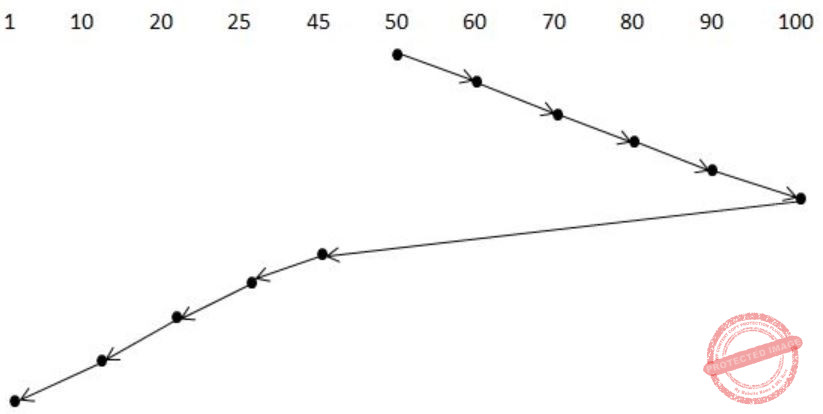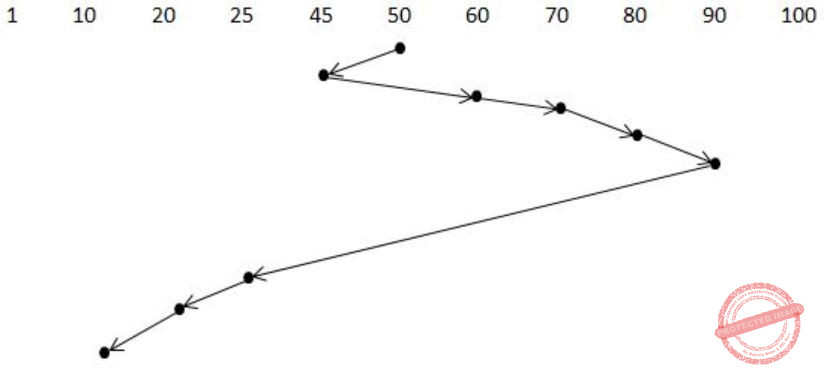## Disk-Scheduling

 Question 1

Consider a storage disk with 4 platters (numbered as 0, 1, 2 and 3), 200 cylinders (numbered as 0, 1, … , 199), and 256 sectors per track (numbered as 0, 1, … 255). The following 6 disk requests of the form [sector number, cylinder number, platter number] are received by the disk controller at the same time:

[120, 72, 2], [180, 134, 1], [60, 20, 0], [212, 86, 3], [56, 116, 2], [118, 16, 1]

Currently head is positioned at sector number 100 of cylinder 80, and is moving towards higher cylinder numbers. The average power dissipation in moving the head over 100 cylinders is 20 milliwatts and for reversing the direction of the head movement once is 15 milliwatts. Power dissipation associated with rotational latency and switching of head between different platters is negligible.

The total power consumption in milliwatts to satisfy all of the above disk requests using the Shortest Seek Time First disk scheduling algorithm is ______ .

 A 85 B 86 C 87 D 88
Operating-Systems       Disk-Scheduling       GATE 2018       Video-Explanation
Question 1 Explanation:
From the above 36 no. question we are getting
→ A storage disk - 4 platters(0, 1, 2 & 3), Cylinder - 200 (0, 1, …, 199) , 256 sector per track.
In the above question the disk requests are given in the form of 〈sector no, cylinder no, platter no〉.
In SSTF (Shortest Seek Time First) Disk Scheduling algorithm, requests having shortest seek time are executed first.
So, the seek time of every request is calculated in advance in queue and then they are scheduled according to their calculated seek time. Head is positioned at 80 and moving towards higher cylinder no.Head Movement in SSTF = (86 – 80) + (86 – 72) + (134 – 72) + (134 – 16) = 200
P1: Power dissipated by 200 movements = 0.2 * 200 = 40 mW
Power dissipated in reversing Head direction once = 15 mW
No. of times Head changes its direction = 3 P2: Power dissipated in reversing Head direction = 3 * 15 = 45 mW
Total power consumption is P1 + P2 = 85 mW
 Question 2

Consider a disk queue with requests for I/O to blocks on cylinders 47, 38, 121, 191, 87, 11, 92, 10. The C-LOOK scheduling algorithm is used. The head is initially at cylinder number 63, moving towards larger cylinder numbers on its servicing pass. The cylinders are numbered from 0 to 199. The total head movement (in number of cylinders) incurred while servicing these requests is ___________.

 A 346 B 347 C 348 D 349
Operating-Systems       Disk-Scheduling       GATE 2016 [Set-1]       Video-Explanation
Question 2 Explanation:
From the question, we got the following information.
I/O to blocks on cylinders 47, 38, 121, 191, 87, 11, 92, 10.
C-LOOK scheduling algorithm is used.
Head is initially at cylinder number 63.
First start from 63
63 → 87 → 92 → 121 → 191 = 24 + 5 + 29 + 70 movements = 128
191 → 10 movements = 181
10 → 11 → 38 → 47 = 1 + 27 + 9 movements = 37
Total = 128 + 181 + 37 = 346
 Question 3

Suppose the following disk request sequence (track numbers) for a disk with 100 tracks is given: 45, 20, 90, 10, 50, 60, 80, 25, 70. Assume that the initial position of the R/W head is on track 50. The additional distance that will be traversed by the R/W head when the Shortest Seek Time First (SSTF) algorithm is used compared to the SCAN (Elevator) algorithm (assuming that SCAN algorithm moves towards 100 when it starts execution) is _________ tracks.

 A 10 B 11 C 12 D 13
Operating-Systems       Disk-Scheduling       GATE 2015 [Set-1]
Question 3 Explanation:
SCAN:= 10 + 10 + 10 + 10 + 10 + 55 + 20 + 5 + 10
= 140
SSTF:= 5 + 15 + 10 + 10 + 65 + 5 + 10 + 10
= 130
= 140 - 130
= 10
 Question 4

Suppose a disk has 201 cylinders, numbered from 0 to 200. At some time the disk arm is at cylinder 100, and there is a queue of disk access requests for cylinders 30, 85, 90, 100, 105, 110, 135 and 145. If Shortest-Seek Time First (SSTF) is being used for scheduling the disk access, the request for cylinder 90 is serviced after servicing ____________ number of requests.

 A 3 B 4 C 5 D 6
Operating-Systems       Disk-Scheduling       GATE 2014 [Set-1]
Question 4 Explanation:90 is serviced after servicing three requests, i.e.,
100 → 105 → 110 → 90
 Question 5

Consider an operating system capable of loading and executing a single sequential user process at a time. The disk head scheduling algorithm used is First Come First Served (FCFS). If FCFS is replaced by Shortest Seek Time First (SSTF), claimed by the vendor to give 50% better benchmark results, what is the expected improvement in the I/O performance of user programs?

 A 50% B 40% C 25% D 0%
Operating-Systems       Disk-Scheduling       GATE 2004
Question 5 Explanation:
There is no improvement in the I/O performance.
→ The better vendor benchmark results doesn't effects the I/O performance.
→ In FCFS (or) SSTF only one choice is to choose for IO from multiple IO's. There is always one I/O at a time.
 Question 6

A Unix-style i-node has 10 direct pointers and one single, one double and one triple indirect pointers. Disk block size is 1 Kbyte, disk block address is 32 bits, and 48-bit integers are used. What is the maximum possible file size?

 A 224 bytes B 232 bytes C 234 bytes D 248 bytes
Operating-Systems       Disk-Scheduling       GATE 2004
Question 6 Explanation:
Maximum size of file = 10 Direct + 1 Single Indirect + 1 Double Indirect + 1 Triple Indirect
= 10 + 28 + (28)2 + (28)3
= 224 Blocks (App)
Size of each block = 210
Maximum file size = 224 × 210 = 234
 Question 7

Using a larger block size in a fixed block size file system leads to:

 A better disk throughput but poorer disk space utilization B better disk throughput and better disk space utilization C poorer disk throughput but better disk space utilization D poorer disk throughput and poorer disk space utilization
Computer-Organization       Disk-Scheduling       GATE 2003
Question 7 Explanation:
While using a larger block size means that contains less number of blocks then that results better throughput. This can be implemented in a fixed block size then the space utilization is not upto the mark. So the statement results better disk throughput but poorer disk space utilization.
 Question 8

Where does the swap space reside?

 A RAM B Disk C ROM D On-chip cache
Computer-Organization       Disk-Scheduling       GATE 2001
Question 8 Explanation:
Swap space is an area on disk that temporarily holds a process memory image.
When memory is full and process needs memory, inactive parts of process are put in swap space of disk.
 Question 9

Which of the following disk scheduling strategies is likely to give the best through put?

 A Farthest cylinder next B Nearest cylinder next C First come first served D Elevator algorithm
Operating-Systems       Disk-Scheduling       GATE 1999
Question 9 Explanation:
Farthest cylinder next - Longest job first
Nearest cylinder next - SSTF
First come first served - FCFS
Elevator - SCAN
SSTF always serves the request of nearest cylinder first. Due to which the necessary movement gets reduced.
 Question 10

The head of a moving head disk with 100 tracks numbered 0 to 99 is currently serving a request at tract 55. If the queue of requests kept in FIFO order is

`  10, 70, 75, 23, 65 `

Which of the two disk scheduling algorithms FCFS (First Come First Served) and SSTF (Shortest Seek Time First) will require less head movement? Find the head movement for each of the algorithms.

 A Theory Explanation.
Operating-Systems       Disk-scheduling       GATE 1995
There are 10 questions to complete.

Register Now